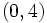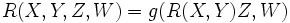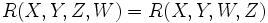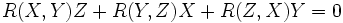# Curvature is antisymmetric in last two variables

(diff) ← Older revision | Latest revision (diff) | Newer revision → (diff)

## Statement

Suppose$M$ is a differential manifold and$g$ is a Riemannian metric or pseudo-Riemannian metric and$\nabla$ is the Levi-Civita connection for$g$. Consider the Riemann curvature tensor$R$ of$\nabla$. In other words,$R$ is the Riemann curvature tensor of the Levi-Civita connection for$g$. We can treat$R$ as a$(0,4)$-tensor:$\! R(X,Y,Z,W) = g(R(X,Y)Z,W)$.

Then:$\! R(X,Y,Z,W) = R(X,Y,W,Z)$.

## Facts used

1. First Bianchi identity: This states that if$R$ is a torsion-free linear connection, then:$R(X,Y)Z + R(Y,Z)X + R(Z,X)Y = 0$.

## Proof

Fill this in later